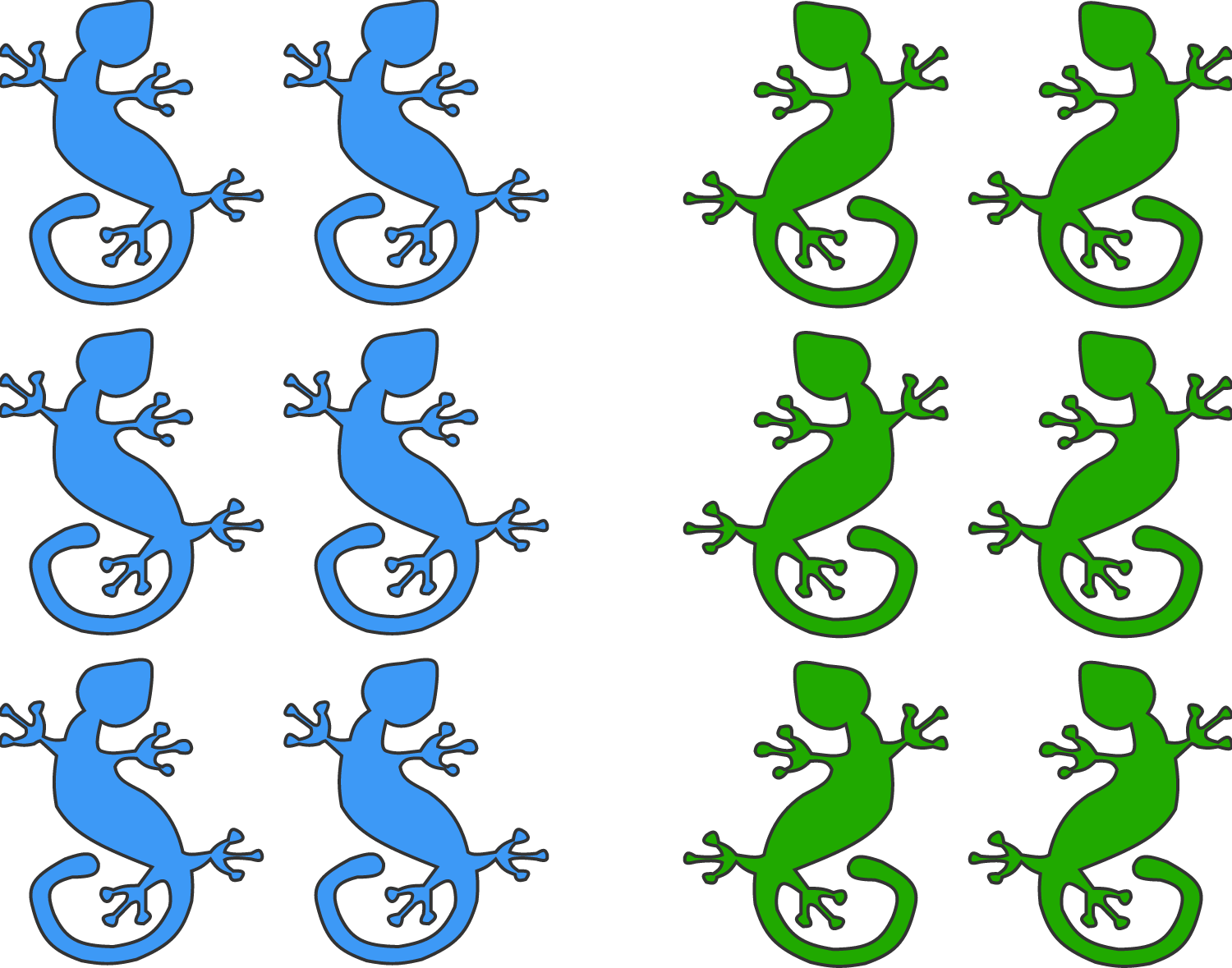# 12 Chameleons

Probability Level 3There are 12 chameleons: 6 are blue, 6 are green.

They randomly form four groups of three. In any group, if there is only one chameleon of a particular color, it changes to the color of the other two. Otherwise, chameleons don't change color.

After this random grouping, if the probability that there will be nine blue chameleons and three green ones is $\dfrac{a}{b}$, where $a$ and $b$ are coprime positive integers, what is $a+b$?

×